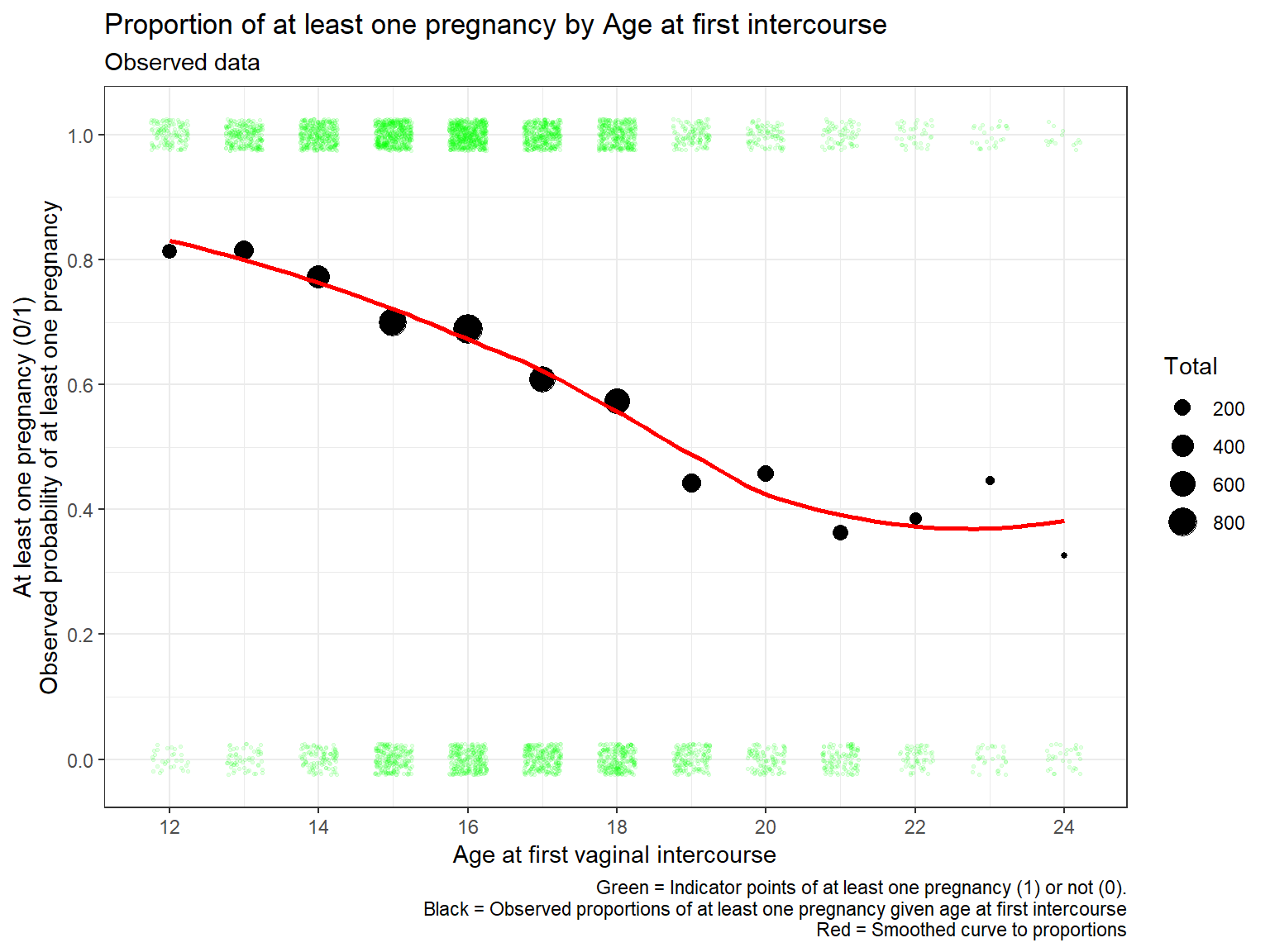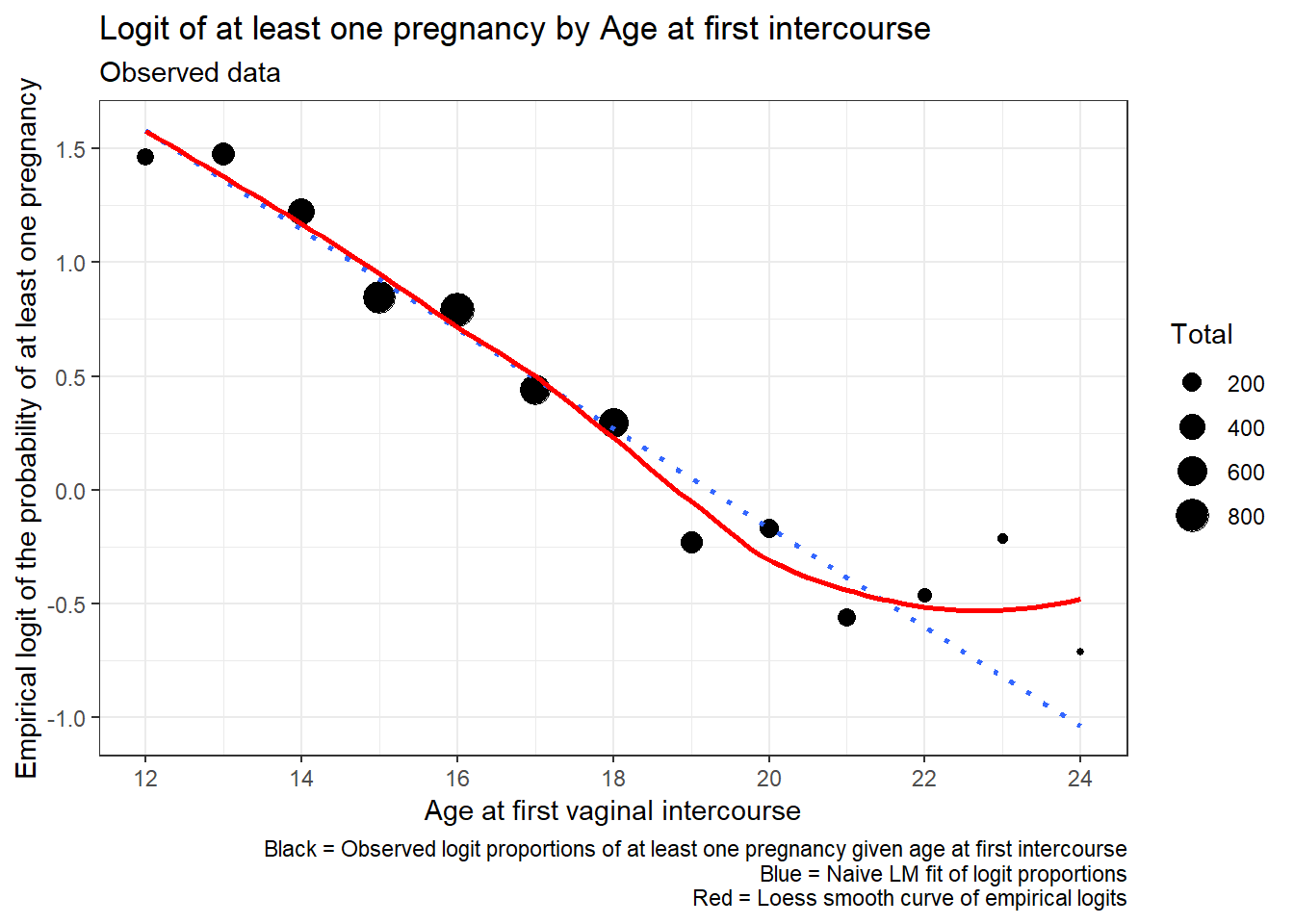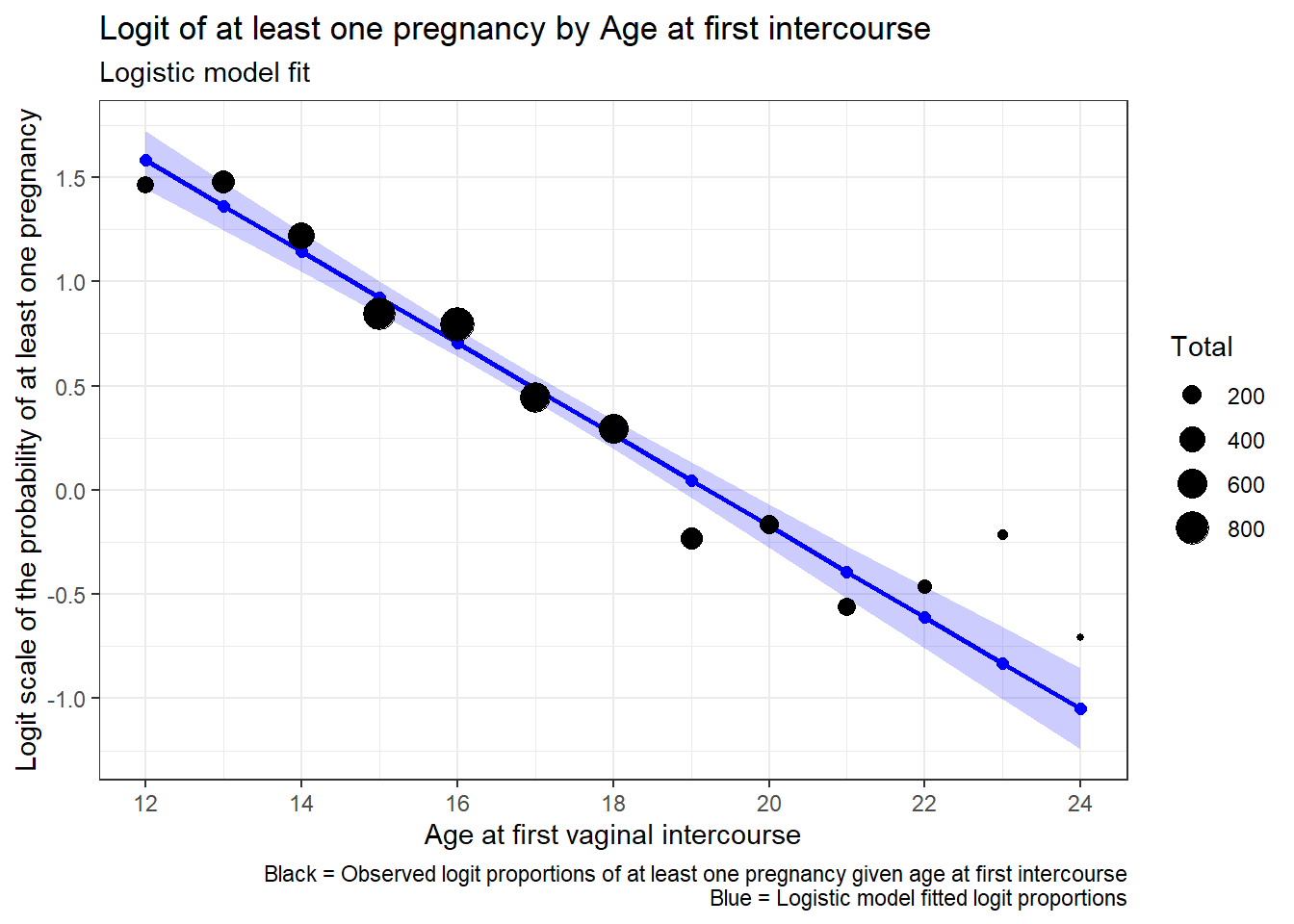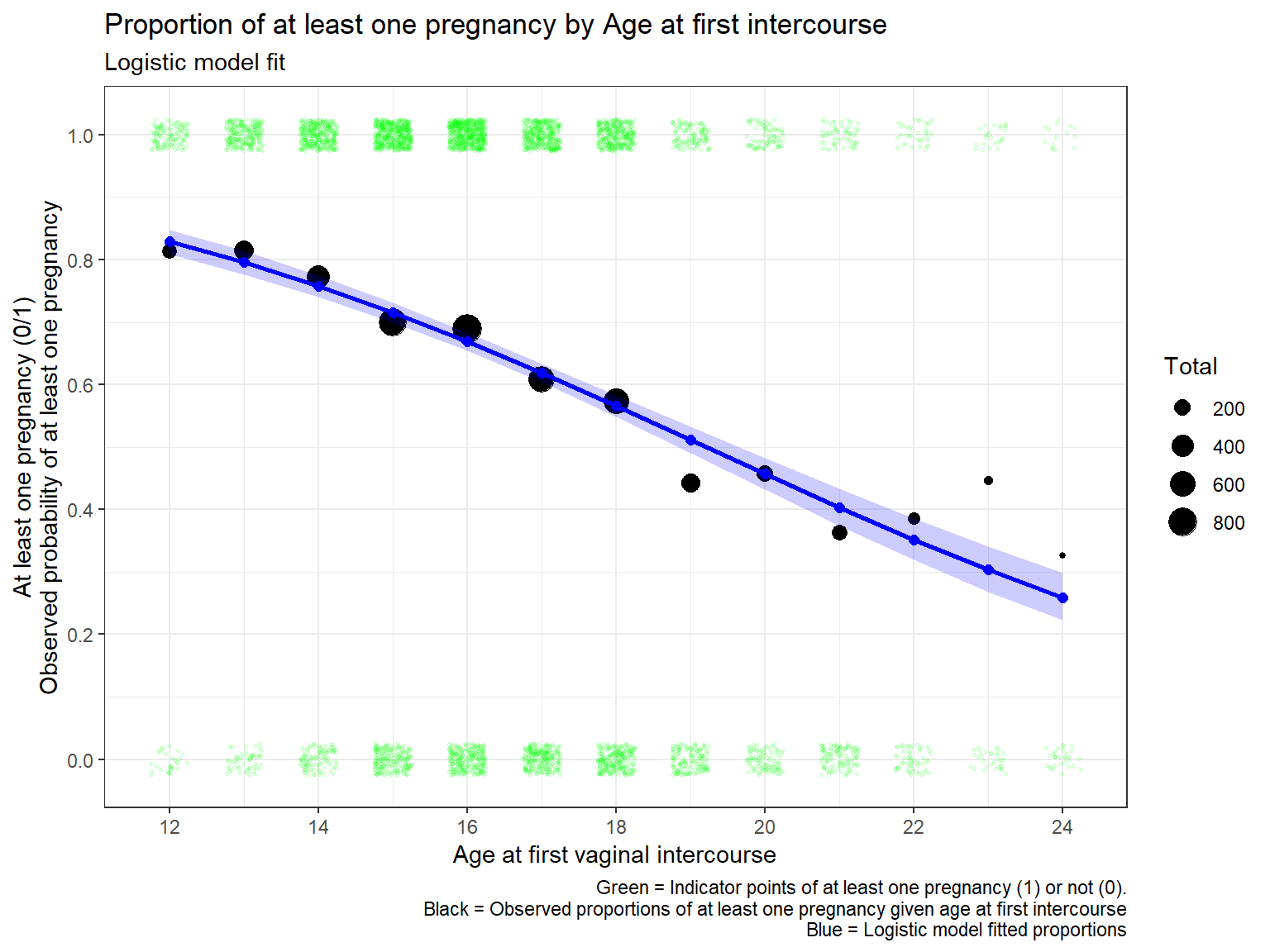Include your answers in this document in the sections below the rubric.

# AddHealth W4: Age at first intercourse vs pregancy

Data from the National Longitudinal Study of Adolescent Health (Add Health), Wave IV.

## Research question

Research question: Is there a relationship between having at least one pregancy and the age at first vaginal intercourse for people in their fertile school years (12–22 years old)? We will define a pregnancy for females as “has been or is pregnant” and for males as “has impregnated partner”.

Intuition: The younger you are when you start having intercourse, the more opportunities you’ll have for pregancy so the more likely it will be that you’ve experienced at least one pregancy. Let’s see if this intuition is observed in the data.

## Data

(Note, if you get in the habit of providing a personal codebook for your work you can give an analysis to someone else to read without having to explain it to them. Everything is documented (also helpful for your future self who doesn’t have as good a memory as you may think you will have).

ADDHEALTH: WAVE4 IN-HOME INTERVIEW CODE BOOK
Wave IV Section 15: Suicide, Sexual Experiences, & Sexually Transmitted Diseases

H4SE7 (AgeAtInt)
7. How old were you the first time you ever had vaginal intercourse?
1-30 years
96 refused
97 legitimate skip
98 don't know

H4TR9 (NPreg)
9. Thinking about all the relationships and sexual encounters you have ever
had, (how many times have you ever been pregnant/how many times have you ever
made a partner pregnant)? Include all pregnancies, whether they resulted in
babies born alive, stillbirth, abortion, miscarriage, or ectopic or tubal
pregnancy. [If Q.7=1] say: Be sure to include your current pregnancy in your
count.
1-19 pregancies
96 refused
98 don't know

Created variables:
EverPreg:   TRUE if NPreg > 0, FALSE if NPreg == 0
EverPreg01:    0 if NPreg > 0,     1 if NPreg == 0

We read the data where missing values have already been coded and create a binary variable for “ever pregnant” (EverPreg).

library(tidyverse)
## -- Attaching packages --------------------------------------- tidyverse 1.3.1 --
## v ggplot2 3.3.5     v purrr   0.3.4
## v tibble  3.1.3     v dplyr   1.0.7
## v tidyr   1.1.3     v stringr 1.4.0
## v readr   2.0.0     v forcats 0.5.1
## -- Conflicts ------------------------------------------ tidyverse_conflicts() --
## x dplyr::lag()    masks stats::lag()
## ## Erik's code to make a separate data file from AH4 data
##
## dat_preg <-
##   select(
##     h4se7
##   , h4tr9
##   ) %>%
##   dplyr::rename(
##     AgeAtInt  = h4se7
##   , NPreg     = h4tr9
##   ) %>%
##   mutate(
##     # assign NA to missing values
##     AgeAtInt  = replace(AgeAtInt, AgeAtInt %in% c(96, 97, 98), NA)
##   , NPreg     = replace(NPreg   , NPreg    %in% c(96, 98)    , NA)
##   )
##
## str(dat_preg)
## summary(dat_preg)
##
##     dat_preg
##   )

dat_preg <-
)
## Rows: 6504 Columns: 2
## -- Column specification --------------------------------------------------------
## Delimiter: ","
## dbl (2): AgeAtInt, NPreg
##
## i Use spec() to retrieve the full column specification for this data.
## i Specify the column types or set show_col_types = FALSE to quiet this message.
dat_preg <-
dat_preg %>%
mutate(
# set as a binary variable, if ever pregnant, then 1, otherwise 0
EverPreg   = (NPreg > 0)  # TRUE or FALSE (1 or 0)
# alternatively, to code as 1 and 0
, EverPreg01 = ifelse(NPreg > 0, 1, 0)  # 1 or 0
) %>%
# remove NAs (the NAs break the logi.hist.plot() below)
# also, with only two variables in the dataset,
#   no analysis can be done if either variable
#   has a missing value for a row observation
na.omit() %>%
# keep people between 12 and 22 years old at first vaginal intercourse
filter(
AgeAtInt >= 12
, AgeAtInt <= 24
)

Summarize observed probability of pregnancy for each age at first vaginal intercourse.

dat_preg_sum <-
dat_preg %>%
group_by(
AgeAtInt
) %>%
summarize(
Success = sum(EverPreg)
, Total   = n()
# estimated proportion of preg for each age group
, p_hat   = Success / Total
, .groups = "drop_last"
) %>%
ungroup()

dat_preg_sum
## # A tibble: 13 x 4
##    AgeAtInt Success Total p_hat
##       <dbl>   <int> <int> <dbl>
##  1       12     131   161 0.814
##  2       13     251   308 0.815
##  3       14     357   462 0.773
##  4       15     503   719 0.700
##  5       16     581   844 0.688
##  6       17     392   644 0.609
##  7       18     342   596 0.574
##  8       19     123   278 0.442
##  9       20      87   190 0.458
## 10       21      65   179 0.363
## 11       22      37    96 0.385
## 12       23      25    56 0.446
## 13       24      15    46 0.326

## Plot the data

### Probability/proportion scale

Plots on the probability scale should follow a sigmoidal curve (a little difficult to see here). Note that the overlayed reference curve (red) is a weighted smoothed curve (loess), not the model fit.

library(ggplot2)
p1 <- ggplot(dat_preg, aes(x = AgeAtInt, y = EverPreg01))
p1 <- p1 + theme_bw()
# data
p1 <- p1 + geom_jitter(width = 0.25, height = 0.025, size = 0.5, alpha = 1/10, colour = "green")
# summaries
p1 <- p1 + geom_point(data = dat_preg_sum, aes(x = AgeAtInt, y = p_hat, size = Total))
p1 <- p1 + geom_smooth(data = dat_preg_sum, aes(x = AgeAtInt, y = p_hat,weight = Total), se = FALSE, colour = "red")  # just for reference
# axes
p1 <- p1 + scale_y_continuous(breaks = seq(0, 1, by = 0.2))
p1 <- p1 + expand_limits(y = c(0, 1))
p1 <- p1 + scale_x_continuous(breaks = seq(0, 100, by = 2))
# labels
p1 <- p1 + labs(
title   = "Proportion of at least one pregnancy by Age at first intercourse"
, subtitle= "Observed data"
, x       = "Age at first vaginal intercourse"
, y       = "At least one pregnancy (0/1)\nObserved probability of at least one pregnancy"
, caption = paste(
"Green = Indicator points of at least one pregnancy (1) or not (0)."
, "Black = Observed proportions of at least one pregnancy given age at first intercourse"
, "Red = Smoothed curve to proportions"
, sep = "\n" # separate by new lines
)
)
print(p1)
## geom_smooth() using method = 'loess' and formula 'y ~ x'### Logit scale

On the logit scale, if points follow a straight line, then we can fit a simple logistic regression model. Note that the overlayed reference straight line (blue) is from weighted least squares (not the official fitted line), and the curve (red) is a weighted smoothed curve (loess). If these two lines are similar, that suggests a straight line on the logit scale is a good fit. Both lines are weighted by the total number of observations that each point represents, so that points representing few observations don’t contribute as much as points representing many observations, thus our decision should not be heavily influcenced by random deviations where there is little data.

# emperical logits
dat_preg_sum <-
dat_preg_sum %>%
mutate(
emp_logit = log((p_hat + 0.5/Total) / (1 - p_hat + 0.5/Total))
)

# plot on logit scale
library(ggplot2)
p1 <- ggplot(dat_preg_sum, aes(x = AgeAtInt, y = emp_logit))
p1 <- p1 + theme_bw()

# summaries
p1 <- p1 + geom_point(aes(size = Total))
p1 <- p1 + stat_smooth(aes(weight = Total), method = "lm", se = FALSE, linetype = 3)  # just for reference
p1 <- p1 + geom_smooth(aes(weight = Total), se = FALSE, colour = "red")  # just for reference

# axes
p1 <- p1 + scale_y_continuous(breaks = seq(-10, 10, by = 0.5))
p1 <- p1 + scale_x_continuous(breaks = seq(0, 100, by = 2))
# labels
p1 <- p1 + labs(
title   = "Logit of at least one pregnancy by Age at first intercourse"
, subtitle= "Observed data"
, x       = "Age at first vaginal intercourse"
, y       = "Empirical logit of the probability of at least one pregnancy"
, caption = paste(
"Black = Observed logit proportions of at least one pregnancy given age at first intercourse"
, "Blue = Naive LM fit of logit proportions"
, "Red = Loess smooth curve of empirical logits"
, sep = "\n" # separate by new lines
)
)
print(p1)
## geom_smooth() using formula 'y ~ x'
## geom_smooth() using method = 'loess' and formula 'y ~ x'(1 p) For the plot above, on the logit scale, does it appear that a straight line fits the data well? If not, what are the features in the data that aren’t being captured by the model, and how concerned are you about any observed deviations from the straight line?

• ???

## Simple logistic regression model

The simple logistic regression model expresses the population proportion $$p$$ of individuals with a given attribute (called the probability of success) as a function of a single predictor variable $$X$$. The model assumes that $$p$$ is related to $$X$$ through $\log \left( \frac{p}{1-p} \right) = \beta_0 + \beta_1 X$ or, equivalently, as $p = \frac{ \exp( \beta_0 + \beta_1 X ) }{ 1 + \exp( \beta_0 + \beta_1 X ) }.$ The logistic regression model is a binary response model, where the response for each case falls into one of two exclusive and exhaustive categories, success (cases with the attribute of interest) and failure (cases without the attribute of interest).

Fit the model.

# For our summarized data (with frequencies and totals for each age)
# The left-hand side of our formula binds two columns together with cbind():
#   the columns are the number of "successes" and "failures".
# For logistic regression with logit link we specify family = binomial,
#   where logit is the default link function for the binomial family.

glm_p_a <-
glm(
cbind(Success, Total - Success) ~ AgeAtInt
, family = binomial
, data   = dat_preg_sum
)

### Deviance statistic for lack-of-fit

Unfortunately, there aren’t many model diagnostics for logistic regression. Visual inspection of the empirical logits is good when you can summarize the data as we’ve done.

One simple test for lack-of-fit uses the deviance statistic. Under the null hypothesis (that you’ll state below), the residual deviance follows a $$\chi^2$$ distribution with the associated degrees-of-freedom.

Below, we calculate the deviance p-value and perform the test for lack-of-fit.

# Test residual deviance for lack-of-fit (if > 0.10, little-to-no lack-of-fit)
glm_p_a$deviance ##  17.36055 glm_p_a$df.residual
##  11
dev_p_val <- 1 - pchisq(glm_p_a$deviance, glm_p_a$df.residual)
dev_p_val
##  0.09765373

(1 p) State the null and alternative hypotheses for lack-of-fit.

• $$H_0:$$ ???

• $$H_A:$$ ???

(1 p) For your preferred model, the deviance statistic is

• D = ??? with
• ??? df, giving
• p-value = ???.

(1 p) What is your conclusion for the model fit?

• ???

## Model visualization and interpretion

The glm() statement creates an object which we can use to create the fitted probabilities and 95% CIs for the population proportions at the ages at first vaginal intercourse. The fitted probabilities and the limits are stored in columns labeled fit_p, fit_p_lower, and fit_p_upper, respectively.

Below I create confidence bands for the model and plot the fit against the data, first on the logit scale to assess model fit and then on the probability scale for interpretation.

# predict() uses all the Load values in dataset, including appended values
fit_logit_pred <-
predict(
glm_p_a
, data.frame(AgeAtInt = dat_preg_sum$AgeAtInt) , type = "link" , se.fit = TRUE ) %>% as_tibble() # put the fitted values in the data.frame dat_preg_sum <- dat_preg_sum %>% mutate( # logit scale values fit_logit = fit_logit_pred$fit
, fit_logit_se    = fit_logit_pred$se.fit , fit_logit_lower = fit_logit - 1.96 * fit_logit_se , fit_logit_upper = fit_logit + 1.96 * fit_logit_se # proportion scale values , fit_p = exp(fit_logit) / (1 + exp(fit_logit)) , fit_p_lower = exp(fit_logit_lower) / (1 + exp(fit_logit_lower)) , fit_p_upper = exp(fit_logit_upper) / (1 + exp(fit_logit_upper)) ) ### Logit scale # plot on logit scale library(ggplot2) p1 <- ggplot(dat_preg_sum, aes(x = AgeAtInt, y = emp_logit)) p1 <- p1 + theme_bw() # MODEL FIT # predicted curve and point-wise 95% CI p1 <- p1 + geom_ribbon(aes(x = AgeAtInt, ymin = fit_logit_lower, ymax = fit_logit_upper), fill = "blue", linetype = 1, alpha = 0.2) p1 <- p1 + geom_line(aes(x = AgeAtInt, y = fit_logit), colour = "blue", size = 1) # fitted values p1 <- p1 + geom_point(aes(y = fit_logit), colour = "blue", size = 2) # summaries p1 <- p1 + geom_point(aes(size = Total)) # axes p1 <- p1 + scale_y_continuous(breaks = seq(-10, 10, by = 0.5)) p1 <- p1 + scale_x_continuous(breaks = seq(0, 100, by = 2)) # labels p1 <- p1 + labs( title = "Logit of at least one pregnancy by Age at first intercourse" , subtitle= "Logistic model fit" , x = "Age at first vaginal intercourse" , y = "Logit scale of the probability of at least one pregnancy" , caption = paste( "Black = Observed logit proportions of at least one pregnancy given age at first intercourse" , "Blue = Logistic model fitted logit proportions" , sep = "\n" # separate by new lines ) ) print(p1)(1 p) For the plot above, comment on the model fit. • ??? ### Probability/proportion scale # plot on probability scale library(ggplot2) p1 <- ggplot(dat_preg, aes(x = AgeAtInt, y = EverPreg01)) p1 <- p1 + theme_bw() # data p1 <- p1 + geom_jitter(width = 0.25, height = 0.025, size = 0.5, alpha = 1/10, colour = "green") # summaries p1 <- p1 + geom_point(data = dat_preg_sum, aes(x = AgeAtInt, y = p_hat, size = Total)) # MODEL FIT # predicted curve and point-wise 95% CI p1 <- p1 + geom_ribbon(data = dat_preg_sum, aes(x = AgeAtInt, y = fit_p, ymin = fit_p_lower, ymax = fit_p_upper), fill = "blue", linetype = 1, alpha = 0.2) p1 <- p1 + geom_line(data = dat_preg_sum, aes(x = AgeAtInt, y = fit_p), colour = "blue", size = 1) # fitted values p1 <- p1 + geom_point(data = dat_preg_sum, aes(y = fit_p), colour = "blue", size = 2) # axes p1 <- p1 + scale_y_continuous(breaks = seq(0, 1, by = 0.2)) p1 <- p1 + expand_limits(y = c(0, 1)) p1 <- p1 + scale_x_continuous(breaks = seq(0, 100, by = 2)) # labels p1 <- p1 + labs( title = "Proportion of at least one pregnancy by Age at first intercourse" , subtitle= "Logistic model fit" , x = "Age at first vaginal intercourse" , y = "At least one pregnancy (0/1)\nObserved probability of at least one pregnancy" , caption = paste( "Green = Indicator points of at least one pregnancy (1) or not (0)." , "Black = Observed proportions of at least one pregnancy given age at first intercourse" , "Blue = Logistic model fitted proportions" , sep = "\n" # separate by new lines ) ) print(p1)## ## Alternative plot. # plot histograms for each condition and fit a logistic curve #library(popbio) popbio::logi.hist.plot( dat_preg$AgeAtInt
, dat_preg\$EverPreg
, logi.mod = 1 # logistic fit
, type = "hist", boxp = FALSE, rug = FALSE
, col = "gray"
, ylabel = "Probability at least one pregnancy (red)"
, ylabel2 = "Frequency"
, xlabel = "Age at first vaginal intercourse"
)